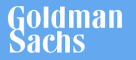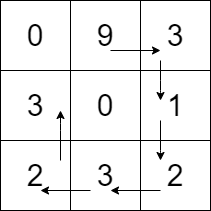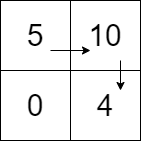New update is available. Click here to update.
Topics

# Path with Maximum Gold

Moderate0/80
Average time to solve is 25m
1 upvote## Problem statement

You’re given a 2 dimensional array 'GRID' of N * M size, representing a Gold mine. Each cell of this grid contains an integer that represents the amount of gold present in that cell.

Your task is to return the maximum amount of gold you can collect using the following conditions:

``````1. Every time you reach a cell, you collect all the gold present in that cell.

2. You can go one step left, right, up, or down from your current cell’s position.

3. You can’t visit the cell which you have already visited.

4. You can’t visit a cell with 0 gold.

5. You can choose any cell to start and stop collecting gold.
``````
Detailed explanation ( Input/output format, Notes, Images )
Constraints:
``````1 <= T <= 5
1 <= N, M <= 10
0 <= GRID[i][j] <=  10 ^ 5

Time limit: 1 sec.
``````
Sample Input 1:
``````1
3 3
0 9 3
3 0 1
2 3 2
``````
Sample Output 1:
``````23
``````
Explanation of Sample Input 1:
``````The optimal way to collect maximum gold is 9->3->1->2->3->2->3, or we start from the 2nd element of the first column, i.e. ‘3’ and follow the path 3->2->3->2->1->3->9, either way, we’ll get the same amount of gold.
``````Sample Input 2:
``````1
2 2
5 10
0 4
``````
Sample Output 2:
``````19
``````
Explanation of Sample Input 2:
``````The optimal way to collect maximum gold is 5->10->4 or 4->10->5; either way, we’ll be able to get the same amount of gold.
``````Console